# ramsey theory

## Multicolour Erdős--Hajnal Conjecture ★★★

Author(s): Erdos; Hajnal

Conjecture   For every fixedand fixed colouring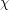ofwithcolours, there existssuch that every colouring of the edges of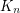contains eithervertices whose edges are coloured according toorvertices whose edges are coloured with at most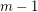colours.

Keywords: ramsey theory

## Geometric Hales-Jewett Theorem ★★

Author(s): Por; Wood

Conjecture   For all integersand, there is an integersuch that for every setof at leastpoints in the plane, if each point inis assigned one ofcolours, then:
\itemcontainscollinear points, or \itemcontains a monochromatic line (that is, a maximal set of collinear points receiving the same colour)

Keywords: Hales-Jewett Theorem; ramsey theory

## Exact colorings of graphs ★★

Author(s): Erickson

Conjecture   For, let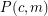be the statement that given any exact-coloring of the edges of a complete countably infinite graph (that is, a coloring withcolors all of which must be used at least once), there exists an exactly-colored countably infinite complete subgraph. Thenis true if and only if,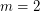, or.

Keywords: graph coloring; ramsey theory

## Monochromatic empty triangles ★★★

Author(s):

If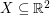is a finite set of points which is 2-colored, an empty triangle is a set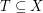withso that the convex hull ofis disjoint from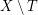. We say thatis monochromatic if all points inare the same color.

Conjecture   There exists a fixed constantwith the following property. Ifis a set ofpoints in general position which is 2-colored, then it has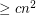monochromatic empty triangles.

Keywords: empty triangle; general position; ramsey theory

## Erdös-Szekeres conjecture ★★★

Author(s): Erdos; Szekeres

Conjecture   Every set ofpoints in the plane in general position contains a subset ofpoints which form a convex-gon.